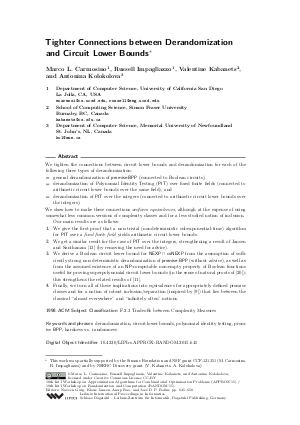Document# Tighter Connections between Derandomization and Circuit Lower Bounds

### Authors Marco L. Carmosino, Russell Impagliazzo, Valentine Kabanets, Antonina Kolokolova## File

LIPIcs.APPROX-RANDOM.2015.645.pdf
• Filesize: 0.52 MB
• 14 pages

## Cite As

Marco L. Carmosino, Russell Impagliazzo, Valentine Kabanets, and Antonina Kolokolova. Tighter Connections between Derandomization and Circuit Lower Bounds. In Approximation, Randomization, and Combinatorial Optimization. Algorithms and Techniques (APPROX/RANDOM 2015). Leibniz International Proceedings in Informatics (LIPIcs), Volume 40, pp. 645-658, Schloss Dagstuhl - Leibniz-Zentrum für Informatik (2015)
https://doi.org/10.4230/LIPIcs.APPROX-RANDOM.2015.645

## Abstract

We tighten the connections between circuit lower bounds and derandomization for each of the following three types of derandomization: - general derandomization of promiseBPP (connected to Boolean circuits), - derandomization of Polynomial Identity Testing (PIT) over fixed finite fields (connected to arithmetic circuit lower bounds over the same field), and - derandomization of PIT over the integers (connected to arithmetic circuit lower bounds over the integers). We show how to make these connections uniform equivalences, although at the expense of using somewhat less common versions of complexity classes and for a less studied notion of inclusion. Our main results are as follows: 1. We give the first proof that a non-trivial (nondeterministic subexponential-time) algorithm for PIT over a fixed finite field yields arithmetic circuit lower bounds. 2. We get a similar result for the case of PIT over the integers, strengthening a result of Jansen and Santhanam [JS12] (by removing the need for advice). 3. We derive a Boolean circuit lower bound for NEXP intersect coNEXP from the assumption of sufficiently strong non-deterministic derandomization of promiseBPP (without advice), as well as from the assumed existence of an NP-computable non-empty property of Boolean functions useful for proving superpolynomial circuit lower bounds (in the sense of natural proofs of [RR97]); this strengthens the related results of [IKW02]. 4. Finally, we turn all of these implications into equivalences for appropriately defined promise classes and for a notion of robust inclusion/separation (inspired by [FS11]) that lies between the classical "almost everywhere" and "infinitely often" notions.
##### Keywords
• derandomization
• circuit lower bounds
• polynomial identity testing
• promise BPP
• hardness vs. randomness

## Metrics

• Access Statistics
• Total Accesses (updated on a weekly basis)
0